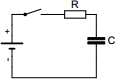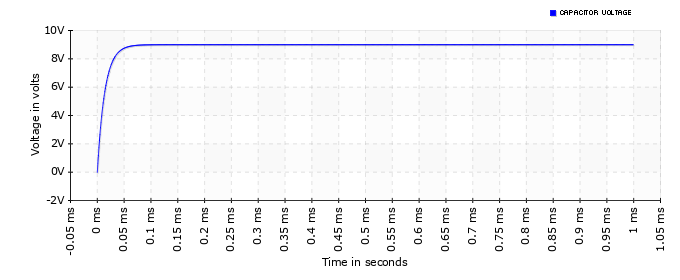# Capacitor charge and discharge calculator

## Calculates charge and discharge times of a capacitor connected to a voltage source through a resistor

 Example 1: Must calculate the resistance to charge a 4700uF capacitor to almost full in 2 seconds when supply voltage is 24V View example Example 2: Must calculate the voltage of a 100nF capacitor after being charged a period of 1ms through 10 kilo-ohm resistor with 5V supply View example Example 3: Must calculate the time to discharge a 470uF capacitor from 385 volts to 60 volts with 33 kilo-ohm discharge resistor View example Example 4: Must calculate the capacitance to charge a capacitor from 4 to 6 volts in 1 millisecond with a supply of 10 volts and a resistance of 1 kilo-ohm View example
 From voltage volts To voltage volts Supply voltage volts Capacitance (C) farads Resistance (R) ohms Time secondsYou may use one of the following SI prefix after a value: p=pico, n=nano, u=micro, m=milli, k=kilo, M=mega, G=gigaResults Peak power of the resistor ~5.785714 watts Peak current ~642.857143 milliamperes